# class ROOT::Math::GaussIntegrator: public ROOT::Math::VirtualIntegratorOneDim

```
User class for performing function integration.

It will use the Gauss Method for function integration in a given interval.
This class is implemented from TF1::Integral().

@ingroup Integration

```

## Function Members (Methods)

public:
 virtual ~GaussIntegrator() void AbsValue(bool flag) virtual double Error() const ROOT::Math::GaussIntegrator GaussIntegrator(const ROOT::Math::GaussIntegrator&) ROOT::Math::GaussIntegrator GaussIntegrator(double absTol = 0, double relTol = 0) virtual double Integral() virtual double Integral(const vector& pts) virtual double Integral(double a, double b) virtual double IntegralCauchy(double a, double b, double c) virtual double IntegralLow(double b) virtual double IntegralUp(double a) virtual int ROOT::Math::VirtualIntegrator::NEval() const ROOT::Math::GaussIntegrator& operator=(const ROOT::Math::GaussIntegrator&) virtual ROOT::Math::IntegratorOneDimOptions Options() const virtual double Result() const virtual void SetAbsTolerance(double eps) virtual void SetFunction(const ROOT::Math::IGenFunction&) virtual void SetOptions(const ROOT::Math::IntegratorOneDimOptions& opt) virtual void SetRelTolerance(double eps) virtual int Status() const virtual ROOT::Math::IntegrationOneDim::Type ROOT::Math::VirtualIntegratorOneDim::Type() const ROOT::Math::VirtualIntegrator ROOT::Math::VirtualIntegrator::VirtualIntegrator() ROOT::Math::VirtualIntegrator ROOT::Math::VirtualIntegrator::VirtualIntegrator(const ROOT::Math::VirtualIntegrator&) ROOT::Math::VirtualIntegratorOneDim ROOT::Math::VirtualIntegratorOneDim::VirtualIntegratorOneDim() ROOT::Math::VirtualIntegratorOneDim ROOT::Math::VirtualIntegratorOneDim::VirtualIntegratorOneDim(const ROOT::Math::VirtualIntegratorOneDim&)
private:
 virtual double DoIntegral(double a, double b, const ROOT::Math::IGenFunction* func)

## Data Members

protected:
 double fEpsAbs Absolute error. double fEpsRel Relative error. const ROOT::Math::IGenFunction* fFunction Pointer to function used. double fLastError Error from the last stimation. double fLastResult Result from the last stimation. bool fUsedOnce Bool value to check if the function was at least called once. static bool fgAbsValue AbsValue used for the calculation of the integral

## Class Charts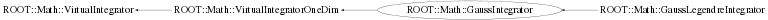## Function documentation

virtual ~GaussIntegrator()
``` Destructor
```
GaussIntegrator(double absTol = 0, double relTol = 0)
``` Default Constructor.
```
void AbsValue(bool flag)
``` Static function: set the fgAbsValue flag.
By default TF1::Integral uses the original function value to compute the integral
However, TF1::Moment, CentralMoment require to compute the integral
using the absolute value of the function.

```
void SetRelTolerance(double eps)
``` Implementing VirtualIntegrator Interface
Set the desired relative Error.
```
`{ fEpsRel = eps; }`
void SetAbsTolerance(double eps)
``` This method is not implemented.
```
`{ fEpsAbs = eps; }`
double Result() const
``` Returns the result of the last Integral calculation.
```
double Error() const
``` Return the estimate of the absolute Error of the last Integral calculation.
```
int Status() const
``` return the status of the last integration - 0 in case of success
```
double Integral(double a, double b)
``` Implementing VirtualIntegratorOneDim Interface

Returns Integral of function between a and b.
Based on original CERNLIB routine DGAUSS by Sigfried Kolbig
converted to C++ by Rene Brun

This function computes, to an attempted specified accuracy, the value
of the integral.

Method:
For any interval [a,b] we define g8(a,b) and g16(a,b) to be the 8-point
and 16-point Gaussian quadrature approximations to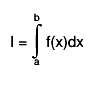$I = #int^{b}_{a} f(x)dx$
and define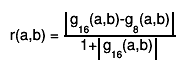$r(a,b) = #frac{#||{g_{16}(a,b)-g_{8}(a,b)}}{1+#||{g_{16}(a,b)}}$
Then,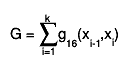$G = #sum_{i=1}^{k}g_{16}(x_{i-1},x_{i})$
where, starting with x0 = A and finishing with xk = B,
the subdivision points xi(i=1,2,...) are given by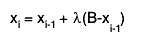$x_{i} = x_{i-1} + #lambda(B-x_{i-1})$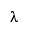$#lambda$
is equal to the first member of the
sequence 1,1/2,1/4,... for which r(xi-1, xi) < EPS.
If, at any stage in the process of subdivision, the ratio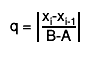$q = #||{#frac{x_{i}-x_{i-1}}{B-A}}$
is so small that 1+0.005q is indistinguishable from 1 to
machine accuracy, an error exit occurs with the function value
set equal to zero.

Accuracy:
The user provides absolute and relative error bounds (epsrel and epsabs) and the
algorithm will stop when the estimated error is less than the epsabs OR is less
than |I| * epsrel.
Unless there is severe cancellation of positive and negative values of
f(x) over the interval [A,B], the relative error may be considered as
specifying a bound on the <I>relative</I> error of I in the case
|I|&gt;1, and a bound on the absolute error in the case |I|&lt;1. More
precisely, if k is the number of sub-intervals contributing to the
approximation (see Method), and if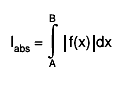$I_{abs} = #int^{B}_{A} #||{f(x)}dx$
then the relation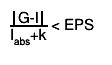$#frac{#||{G-I}}{I_{abs}+k} < EPS$
will nearly always be true, provided the routine terminates without
printing an error message. For functions f having no singularities in
the closed interval [A,B] the accuracy will usually be much higher than
this.

Error handling:
The requested accuracy cannot be obtained (see Method).
The function value is set equal to zero.

Note 1:
Values of the function f(x) at the interval end-points A and B are not
required. The subprogram may therefore be used when these values are
undefined

```
double Integral()
``` Returns Integral of function on an infinite interval.
This function computes, to an attempted specified accuracy, the value of the integral: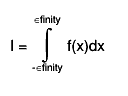$I = #int^{#infinity}_{-#infinity} f(x)dx$
Usage:
In any arithmetic expression, this function has the approximate value
of the integral I.

The integral is mapped onto [0,1] using a transformation then integral computation is surrogated to DoIntegral.

```
double IntegralUp(double a)
``` Returns Integral of function on an upper semi-infinite interval.
This function computes, to an attempted specified accuracy, the value of the integral: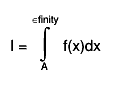$I = #int^{#infinity}_{A} f(x)dx$
Usage:
In any arithmetic expression, this function has the approximate value
of the integral I.
- A: lower end-point of integration interval.

The integral is mapped onto [0,1] using a transformation then integral computation is surrogated to DoIntegral.

```
double IntegralLow(double b)
``` Returns Integral of function on a lower semi-infinite interval.
This function computes, to an attempted specified accuracy, the value of the integral: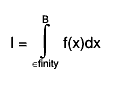$I = #int^{B}_{#infinity} f(x)dx$
Usage:
In any arithmetic expression, this function has the approximate value
of the integral I.
- B: upper end-point of integration interval.

The integral is mapped onto [0,1] using a transformation then integral computation is surrogated to DoIntegral.

```
void SetFunction(const ROOT::Math::IGenFunction& )
``` Set integration function (flag control if function must be copied inside).
\@param f Function to be used in the calculations.

```
double Integral(const vector<double>& pts)
``` This method is not implemented.
```
double IntegralCauchy(double a, double b, double c)
``` This method is not implemented.
```
void SetOptions(const ROOT::Math::IntegratorOneDimOptions& opt)
``` set the options
```
double DoIntegral(double a, double b, const ROOT::Math::IGenFunction* func)
```      Integration surrugate method. Return integral of passed function in  interval [a,b]
Derived class (like GaussLegendreIntegrator)  can re-implement this method to modify to use
an improved algorithm

```